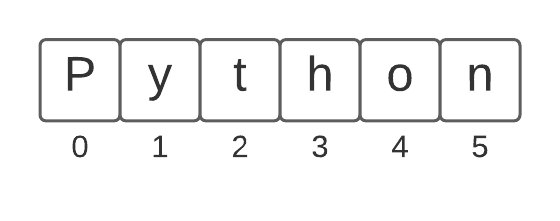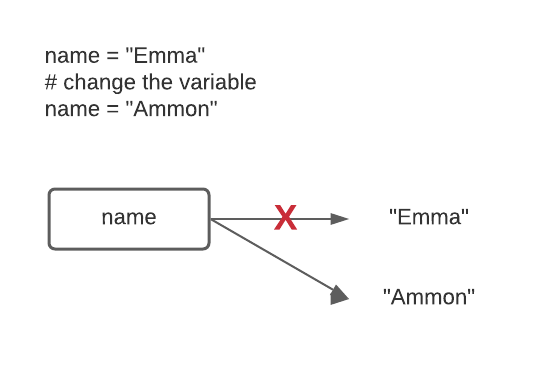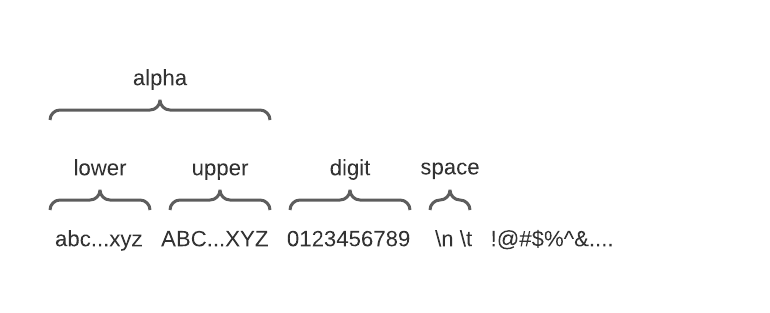Computer Science

# Strings

• we have used strings previously
• a string is a sequence of characters
• strings, integers, floats, booleans are all called data types
• can use single or double quotes
``````def say_hello(name):
print(f"Hello, {name}.")

say_hello("Emma")

bit.paint('blue')``````

## `len()` function

• returns the number of characters in a string
• try these in the interpreter
``````len('Hello')
s = 'Hello'
len(s)
len('x')
len('')``````
• `''` or `""` represent the empty string, length zero
• when you are working with strings, make sure your code works with empty strings!

### We count from zero!

• also called zero-based indexing
• pixels in an image started with (0, 0)
• if the width of an image is 800, it goes from 0 to 799
• we index strings the same way!### indexing strings

• we can access each character in the string using square brackets
• be careful to use a valid index — from 0 to len-1
• try these in the interpreter
``````s = 'Python'
s
s
s
s
s``````

## strings are immutable (they can’t be changed)

• try these in the interpreter
``````s = 'Python'
s
s = 'X'``````

• we can use the `+` operator to combine two strings into a new, bigger string
• does not change the original strings
• try these in the interpreter
``````a = 'Hello'
b = 'there'
a + b
a
a + " " + b``````

### changing variables

• when you change a variable, it now “points” to the new value### appending to a string

• can use the `+` operator
• try these in the interpreter
``````name = "Emma"
name = name + '!'
name
name = "Julie"
name += '!'
name += '!'
name += '!'
name``````

## looping over strings

``````name = "Julie"
for i in range(len(name)):
print(name[i])``````

### write a `double_char()` function

• loop over each character in the string
• add two copies of each character to the result
• return the result
``````def double_char(s):
""" Take a string and return the same thing but with every character doubled."""
result = ""
for i in range(len(s)):
# try uncommenting
print(i,result)
result = result + s[i] + s[i]
print(i, result)
return result

print(double_char("crazy"))``````
``````    0
0 cc
1 cc
1 ccrr
2 ccrr
2 ccrraa
3 ccrraa
3 ccrraazz
4 ccrraazz
4 ccrraazzyy
ccrraazzyy``````

### You will see this pattern a lot

2. add characters to the string
3. return the result
``````def double_char(s):
""" Take a string and return the same thing but with every character doubled."""
result = ""
for i in range(len(s)):
# try uncommenting this line
# print(s[i])
result = result + s[i] + s[i]
return result

print(double_char("crazy"))

``````

## String tests

• `s.isalpha()` - True if all characters in s are alphabetic

• `s.isdigit()` - True if all characters in s are digits ‘0’, ‘1’, .. ‘9’

• `s.isalnum()` - True if all characters are s are alphabetic or numeric

• `s.isspace()` - True if all characters in s are whitespace characters — space, tab, newline### Try these string tests in the Python interpreter

``````'a'.isalpha()
'abc'.isalpha()
'Z'.isalpha()
'\$'.isalpha()
'@'.isalpha()
'9'.isdigit()
' '.isspace()
'Ω'.isalpha()
'   \t  \n'.isspace()``````

## Function patterns

### if pattern

• write an `alpha_only()` function

• returns a new string that has only the alphabetical characters in it

``````def alpha_only(s):
result = ''
for i in range(len(s)):
if s[i].isalpha():
result += s[i]
return result

alpha_only("I'm 91. You call me old??! I'm wise and experienced!")``````
``    'ImYoucallmeoldImwiseandexperienced'``

### if else pattern

• write a `str_dx()` function
• return a string where every digit becomes ‘d’ and every other character becomes ‘x’
``````def str_dx(s):
result = ''
for i in range(len(s)):
if s[i].isdigit():
result += 'd'
else:
result += 'x'
return result

str_dx("I'm 91. You call me old??! I'm wise and experienced!")``````
``    'xxxxddxxxxxxxxxxxxxxxxxxxxxxxxxxxxxxxxxxxxxxxxxxxxxx'``

### if and not pattern

• write an `only_nondigits()` function
• return a string with only the non-digits
``````def only_nondigits(s):
result = ''
for i in range(len(s)):
if not s[i].isdigit():
result += s[i]
return result

only_nondigits("I'm 91. You call me old??! I'm wise and experienced!")``````
``    "I'm . You call me old??! I'm wise and experienced!"``

### early return pattern

• write a `has_alpha()` function
• returns true if there is at least one alphabetic character in the string
``````def has_alpha(s):
for i in range(len(s)):
if s[i].isalpha():
return True
return False

print(has_alpha('45#a8e'))
print(has_alpha('45^#)-'))``````
``````    True
False``````

## Doctests

• can put these into your docstring
• look exactly like what you would see in the Python console
• first line is the test, second line is the result
• can run them from Pycharm
``````>>> has_alpha('45#a8e')
True
>>> has_alpha('45^#)-')
False``````

### Doctests example

• in Pycharm, right click on the function name and select `Run ‘Doctest has_alpha
• trying putting in a bug and see what happens
``````def has_alpha(s):
"""
Returns true if there are any alphabetic characters in the string, false otherwise.

:param s: a string
:return: True if there are alphabetic characters in s, otherwise False
>>> has_alpha('45#a8e')
True
>>> has_alpha('45^#)-')
False
"""
for i in range(len(s)):
if s[i].isalpha():
return True
return False``````

• Use divide-and-conquer to break the problem down into smaller parts
• Work on one function at a time
• Write documentation first
• Write doctests second
• Now write code
• Get it to past easy tests first
• Then check “edge” cases, like an empty string
• Get the function to pass all tests before moving on to the next function
• Your life will be much easier!

### Avoid

• Writing a bunch of code with no documentation and no tests and then “trying” it to see if it will work